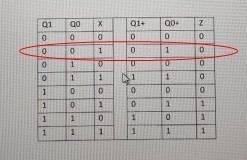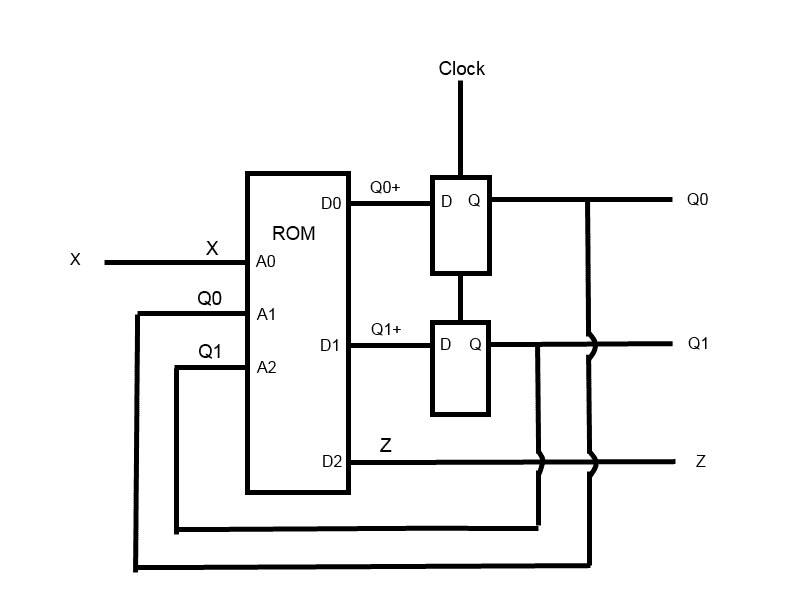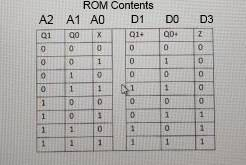# Digital Logic Help Needed

I cannot figure out where the values from Q1+ and Q0+ are coming from. What truth table are these coming from?? I would greatly appreciate any help. Thank you.

#### Attachments

•20160504_092327.jpg
28.6 KB · Views: 501

CWatters
Homework Helper
Gold Member
With reference to the truth table you posted...

The left hand side of the truth table lists the "current state" and any inputs (eg X).
The right hand side of the truth table lists the "next state" and any outputs (eg Z).

The "+" sign basically means "after the next clock". So the column headed Q1+ is telling you what Q1 will become after the next clock.

So for example if we look at the second line of the truth table it is telling us that..

If the current state is Q1=0, Q0=0 and X=1 then the next state after the clock will be Q1=0, Q0=1 and Z=0CWatters
Homework Helper
Gold Member
PS Here is one way to build the state machine in your problem..Two D-Type latches are used to hold the state bits. A very small Read Only Memory (ROM) is used to implement the truth table. Q0, Q1 and X are used as address bits to the ROM. The ROM data outputs D0, D1, D2 are used to generate the "next state" Q0+ and Q1+ and the output Z.You don't have to use a ROM to implement this. You could work out how to generate (for example) Q0+ from Q1,Q0 an X using logic gates instead.

One thing missing from my circuit is a reset pin. Typically this would reset both latches to 00 (or some other known state) when power is first applied.